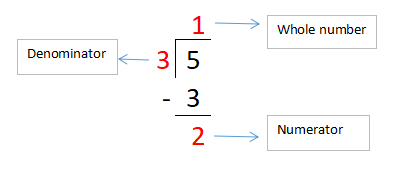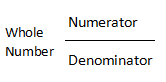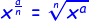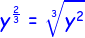# Top 15 Examination Question And Answer On Mathematics For Secondary School Student

Mathematics (from Grek μάθημα máthēma, “knowledge, study, learning”) is the study of such topics as quantity, structure, space, and change. It has no generally accepted definition. How will you answer, top 15 examination question and answer on mathematics for secondary school student?

## 1.Question:

15 is 25 percent of what number?

15 is 25% of 60.

In general, to solve percent problems, we can use the following rule:

• If x is y% of z, then x = (y% in decimal form) × z

Suppose you are helping a charity that is collecting bicycles to give to kids that can’t afford bikes. So far, you’ve collected 15 bicycles, and the head of the charity says that this is 25% of the total number of bikes that they need. In order to figure out how many bikes that they need, we would need to know what number that 15 is 25% off.

If we let this number be z, then we have the statement 15 is 25% of z and we can use our rule to convert this to our percent equation.

• 15 is 25% of z is equivalent to 15 = (25% in decimal form) × z

To write any percent in decimal form, we just move the decimal point two units to the left and remove the percent symbol, so 25% in decimal form is 0.25. Therefore, our percent equation is 15 = 0.25z. Now we just solve this equation for z by dividing both sides by 0.25.

• 15 / 0.25 = 0.25z / 0.25
• 60 = z

We get that z = 60. Therefore, the total number of bikes that the charity needs is 60 bikes. Plugging z = 60 back into our statement gives that 15 is 25% of 60.

## 2.Question:

Find the geometric mean between 12 and 16.

The geometric mean between 12 and 16 is approximately 13.8564.

In general, to find the geometric mean of a set of n numbers, we multiply all the numbers together, and then take the nth root of the result. Therefore to find the geometric mean of the set of number {12,16}, we multiply 12 by 16 and then take the square root of the result.

• 12 × 16 = 192
• √(192) ≈ 13.8564

We get that the geometric mean of 12 and 16 is approximately 13.8564.

## 3.Question:

What is 10% of 1 billion?

10% of 1 billion is 100 million.

Suppose the national lottery has gotten up to 1 billion dollars and if somebody wins, the payout is done in such a way that the winner receives 10% of the total amount won each year for 10 years. To determine how much the winner would get each year, we need to calculate 10% of 1 billion.

In general, to find x% of y, we multiply y by the decimal form of x%. To put x% in decimal form, we move the decimal in x two units to the left and remove the percent symbol.

Therefore, to find 10% of 1 billion, we multiply 1 billion by 10% in decimal form. To put 10% in decimal form, we move the decimal point in 10% two units to the left and then remove the percent symbol to get 0.10. Now, we just multiply 1 billion, or 1,000,000,000 by 0.10.

• 1,000,000,000 × 0.10 = 100,000,000

We get that 10% of 1,000,000,000, or 1 billion, is 100,000,000, or 100 million. Therefore, the winner of the lottery would receive 100 million dollars each year for 10 years.

## 4.Question:

What is 3.75 as a mixed number?

The decimal 3.75 would be 3 75/100 as a mixed number. In lowest terms, it would be 3 3/4.

To change a decimal to a mixed number, think about place value. The ‘3’ is in the one’s place, so it stands for 3 ones. This is your whole number in the mixed number.

The numbers to the right of the decimal place will be the fraction in your mixed number. The ’75’ means ’75 hundredths.’ This is written as 75/100 in fraction form. 75/100 is not in simplest terms; it can be reduced. To reduce it, divide both 75 and 100 by 25, which is their greatest common factor. This gives you the fraction 3/4.

## 5.Question:

What is the prime factorization of 56?

The prime factorization of 56 is 2 * 2 * 2 * 7. This can also be written with exponents as 2^3 * 7.

When you find the prime factorization, you’re breaking a number down into factors until all of the factors are prime and cannot be broken down any farther.

Here’s the process:

• Break down 56 into 2 * 27.
• Then break down 27 into 2 * 14.
• Next, break down 14 into 2 * 7.

You can see that the prime numbers in this factorization are 2 * 2 * 2 * 7, or 2^3 * 7.

## 6.Question:

What is the square root of 250?

The square root of 250 is 5√10 or approximately 15.81139.

Let’s review the factors of 250. They are: 1,2, 5, 10, 25, 50, 125 and 250.

Let’s check if any of these factors is the square root of 250, by multiplying each by itself: 1 x 1 =1; 2 x 2 = 4; 5 x 5 = 25; 10 x 10 = 100; 25 x 25 = 625; 50 x 50 = 2500; 125 x 125 = 15,625; 250 x 250 =62,500. As you can see, none of these products equals 250, so none of these factors is the square root of 250.

Hence, there is no rational square root. But, we can simplify this square root by noting that one of the factors, 25, has a square root of 5. So:

√250 = √25 x √10 = 5√10

## 7.Question:

What is 0.64 as a fraction?

The decimal 0.64 is equivalent to the fraction 64/100.

To write this fraction, read the decimal. The decimal is ’64 hundredths’. You know this because the ‘4’ is in the hundredths place. To write this as a fraction, the 64 is the numerator and the 100 is the denominator. So, ’64/100′ is equivalent to ’64 hundredths’.

If you need to write the fraction in simplest form, you’ll need to divide the numerator and denominator of the fraction by the greatest common factor of 64 and 100. The GCF would be 4. Divide 64 by 4 and you get 16; divide 100 by 4 and you get 25. This makes your new fraction 16/25.

16/25 is equivalent to 64/100, and both are equivalent to 0.64.

## 8.Question:

What is 5 divided by 3 in fraction form?

The answer is 5 / 3 or 1 2/3

5 divided by 3 can be written simply as 5 / 3.

To make it into a mixed number, we divide the numbers by using a short division in order to find out how many times one number can go into the other and what is left over when we’re done (if anything).As shown in the illustration, we see that our whole number is 1 and the remainder is 2.

Now, we make a guideline as shown in the second illustration.The remainder becomes a numerator because there is still 2 parts leftover of 3.

Thus, our mixed number answer is,or an improper fraction of 5 / 3.

## 9.Question:

How many pennies are in a million dollars?

There are 100,000,000 pennies in one million dollars.

To find the number of pennies in a million dollars, think first about the number of pennies in one dollar. There are 100 pennies in one dollar.

Because there are 100 pennies in one dollar, to find the number of pennies in one million dollars, you’d need to multiply 100 (number of pennies in one dollar) by one million (the number of dollars you have).

100 x 1,000,000 = 100,000,000

One hundred times one million equals one hundred million, or 100,000,000. So, there are 100,000,000 pennies in a million dollars.

## 10.Question:

What number is equal to its opposite?

The number that is equal to its opposite is 0.

To see why this is, let’s consider some properties of opposite numbers.

• Opposite numbers lie the same distance in opposite directions of the origin on a number line.
• If we add a number to its opposite, we get 0.

Consider the first property. For a number and its opposite to be equal, it would have to be a number that is the same distance from the origin in opposite directions on the number line. The only number that would satisfy this is 0. Any number other than zero would be x units in one direction from 0 on the number line, so its opposite would have to be x units in the other direction from 0 on the number line. Therefore, the only number that satisfies this property is 0.

Now, think about the second property. If we add a number to its opposite, we get 0. For example,

• 2 + (-2) = 0

Remember we’re looking for a number and its opposite that are equal to each other. Rather than looking for x and –x, we’re actually looking for only ONE number: x. Therefore, x + x must equal 0 giving the following equation.

• x + x = 0

We can solve this equation for x to find a number that is equal to its opposite.

• x + x = 0
• Simplify the left-hand side of the equation.
• 2x = 0
• Divide both sides of the equation by 2.
• 2x / 2 = 0 / 2
• Simplify.
• x = 0

We get that x = 0, so we see that 0 is the number that is equal to its opposite.

If you’re still not convinced, then just consider 0 and its opposite, -0. Multiplying 0 by a negative doesn’t change the value of 0. That is,

• -0 = (-1)(0) = 0

Therefore, we see again that 0 is equal to its opposite.

## 11.Question:

How to write an expression in radical form

Given an algebraic expression involving exponents, we can write it in a radical form based on the fact that x(a/n) is equivalent to the nth root of xa.That is, we can write an expression in a radical form using the following steps.

1. Use rules of exponents to get the expression in the form x(a/n), where x can be a number, variable, or algebraic expression.
2. Use the rule to rewrite x(a/n) as the nth root of xa.

For example, consider the algebraic expression (y2)(1/3). We can rewrite this in a radical form using our steps. First, we need to get it in the form x(a/n). We can use the following rule of exponents to do this.

• (xa)b = xab

Therefore, we can rewrite our expression by multiplying the exponents 2 and 1/3.

• (y2)(1/3) = y2 × (1/3) = y(2/3)

Now, we just use our rule for converting to radical form to rewrite y(2/3) as the third root of y2.This gives us our original expression in radical form.

## 12.Question:

If we have 300 centiliters, we would have how many liters?

If we have 300 centiliters, then we would have 3 liters.

To find out how many liters is equal to 300 centiliters, we need to convert 300 centiliters to liters. We do this using the following conversion fact:

• 1 centiliter = 1/100 of a liter

Since 1 centiliter is 1/100 of a liter, 300 centiliters would be 300 times 1/100 of a liter, so we convert 300 centiliters to liters by multiplying 300 by 1/100.

• 300 × (1/100) = 300/100 = 3

We see that 300 centiliters are equivalent to 3 liters.

## 13.Question:

What unit of measurement comes after kilometers?

In the Metric System, the units of measurement that comes after kilometers are megameters. One megameter is equivalent to one million meters.

The basic unit of length in the Metric System is the meter. We use different metric prefixes to indicate a specific amount of meters. Some of those prefixes are as follows:

• giga = one billion
• mega = one million
• kilo = one thousand
• hecto = one hundred
• deka = ten
• BASE UNIT (NO PREFIX) = one
• deci = one tenth
• centi = one hundredth
• milli = one thousandths
• micro = one millionth
• nano = one billionth

For instance, since ‘kilo’ is the prefix of a kilometer, we have that one kilometer is equal to one thousand meters.

From this list of prefixes, we see that the unit of measurement that comes after kilometers in the metric system is megameters. Because the prefix ‘mega’ means one million, we have that one megameter is equivalent to one million meters.

## 14.Question:

How many nanoseconds in a second?

Noting that for every 1 nanosecond, there are 1 x 10-9 seconds, we can convert any number of seconds to an equivalent number of nanoseconds. i.e. 1 second x 1 nanosecond / (1 x 10-9) seconds = 1 x 109 nanoseconds. In other words, there are 1 x 109 nanoseconds in 1 second.

## 15.Question:

What is the top number of a fraction called?